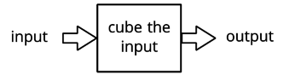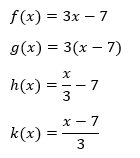# A.4.4.2 Four Functions

Topic:
Functions

Here is a "function machine"This a function takes any input and cubes it to generate the output. Find the output when the inputs are 0, 1, 3, and x, use function notation and the function g.

Some functions have a specific rule for getting its output. The rule can be described in words (like “cube the input”) or with expressions (such as x^3). Now we'll look at some rules expressed in both ways.

Here are descriptions and equations that represent four functions.A. To get the output, subtract 7 from the input, then divide the result by 3. B. To get the output, subtract 7 from the input, then multiply the result by 3. C. To get the output, multiply the input by 3, then subtract 7 from the result. D. To get the output, divide the input by 3, and then subtract 7 from the result. Match each equation with a verbal description that represents the same function. Record your results.

The expressions for functions f and g both involve multiplying by 3 and subtracting 7. How are they different?

For one of the functions, when the input is 6, the output is -3. Which is that function: f, g, h, or k? Explain how you know.

Which function value—f(x), g(x), h(x), or k(x)—is the greatest when the input is 0? What about when the input is 10?